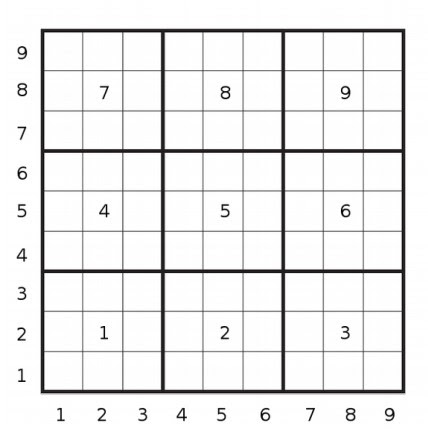# Daily Challenge #70

Each small square is 30x30, and a big one is 90x90.
The program should ask for x and y coordinates and the program should return three numbers. The first one the square x is in, the second y, and the third the big square they are both in.Examples:

``````function(128,230) => (8 5 8)
function(180,180) => (6 6 5)
function(181,181) => (7 7 9)
``````

Sorry, kinda forgot that I had to write today’s DC.

Have fun!

3 Likes

Добар задатак мај дјуд

1 Like

First, I do not understand the thing with the different squares.

Aand also, what happens if it is on a corner, likeThen what’s this

x is 128, y is 230

I just don’t understand how come 128 will be x

Just an example input number.

I know it is a input number, but is the x coordinate the phone coordinate?

Ummm no. I think you have to look at it again. The image is the point.

OHHH i finally understand. I thought small squares mean the 3x3 square before lol

1 Like